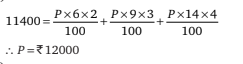# Mathematical Aptitude - Interest

>>>>>>>>Interest

• A

Rs 12000• B

Rs 11400• C

Rs 11000• D

Rs 10000• Option : A
• Explanation :• A

Rs 695.80, 11.11%• B

Rs 625, 12.50%• C

Rs 656.10, 11.11%• D

Rs 686, 12.50%• Option : C
• Explanation : Hint: First calculate that Rs 900 becomes Rs 1000 in fourth year. Calculate rate of interest by application of simple interest formula. Thereafter principal amount can also be calculated.

• A

Rs 56• B

Rs 62• C

Rs 70• D

Rs 76• A

Rs 29000• B

Rs 30000• C

Rs 31000• D

Rs 28000• Option : A
• Explanation : Hint: First initial R can be calculated, and then 3% added to it.

• A

Rs 0• B

Rs 150• C

Rs 280• D

Rs 320• Option : A
• Explanation : We can solve this question directly.
The simple interest amount is same in both cases.
15 × 4 = 20 × 3
Related Quiz.
Interest